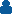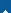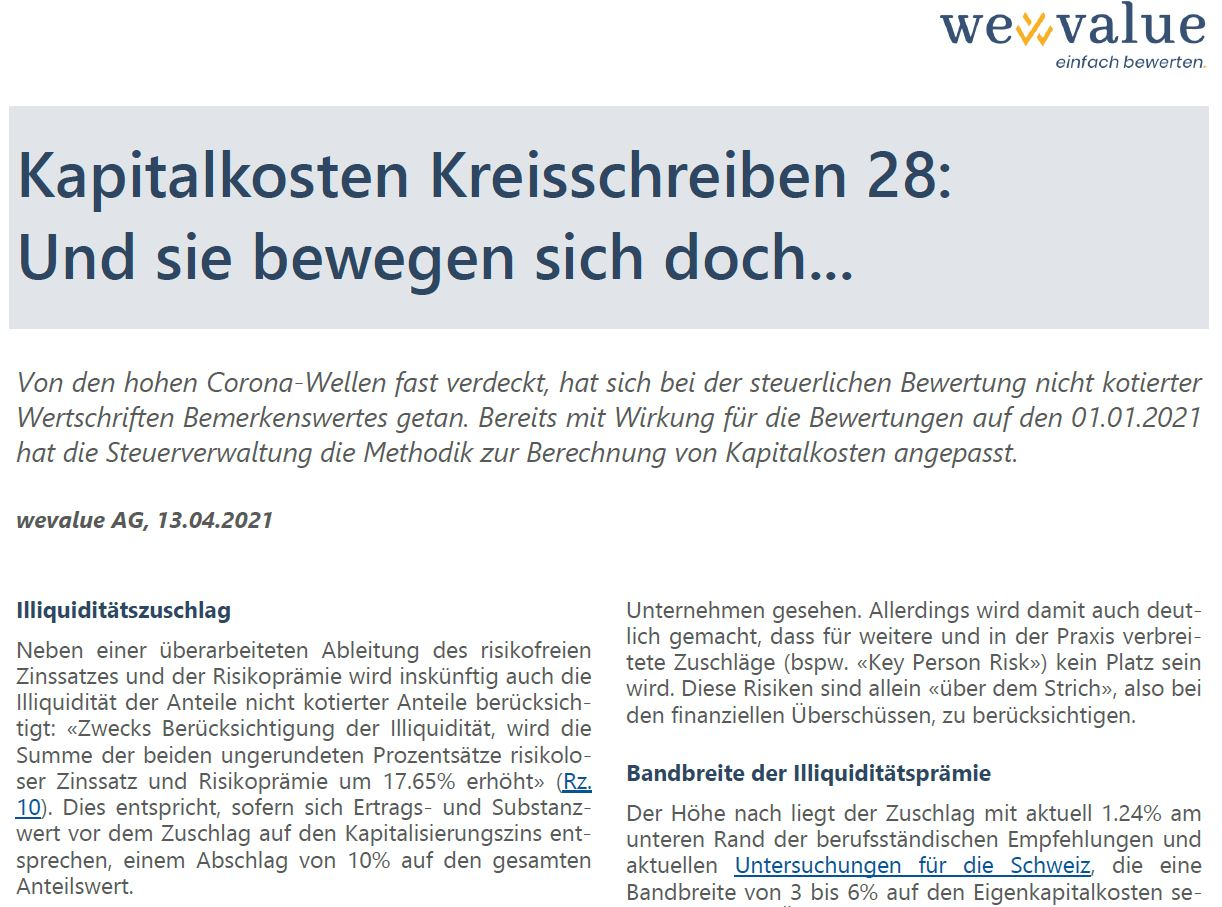# Cost of capital circular 28

13.04.2021wevalue AGAll, Specialist articles##### And yet they move…

Almost obscured by the high Corona waves, remarkable things have happened in the tax valuation of unlisted securities. Already with effect for the valuations on 01.01.2021, the tax administration has adjusted the methodology for calculating the cost of capital.

In addition to a revised derivation of the risk-free interest rate and the risk premium, the illiquidity of the units of unlisted units will also be taken into account in the future: “For the purpose of taking illiquidity into account, the sum of the two unrounded percentages risk-free interest rate and risk premium shall be increased by 17.65%” (margin note 10). This corresponds to a discount of 10% on the total unit value, provided that the income value and net asset value are the same before the capitalization rate is added.

###### Example

Income to be capitalized 1,000, capitalization rate (old) 7.00%, income value 14,286, net asset value assumed to be the same (14,286), results in a unit value of 14,286. If illiquidity is now taken into account with an increase of 17. 65%, this results in a new capitalization rate of 8.24% (7.00% x 1.1765), a new capitalized earnings value of 12,136 and – with unchanged net asset value – a unit value of 12,853 (2/3 weighting of capitalized earnings value and 1/3 weighting of net asset value).

Read the whole article here (in German).

<< back
forward >>
wevalue – the solution for